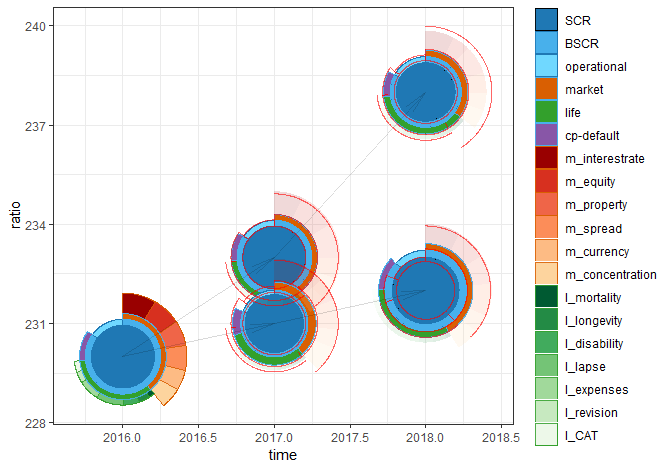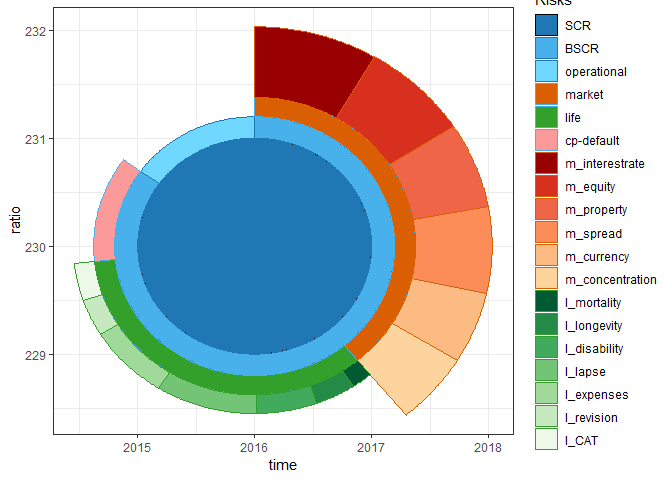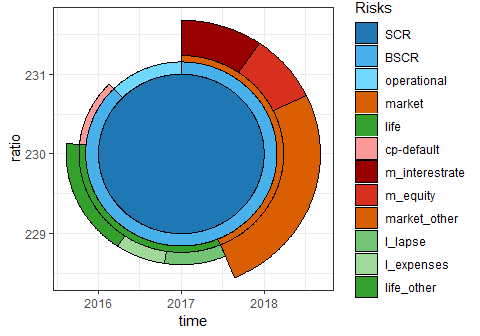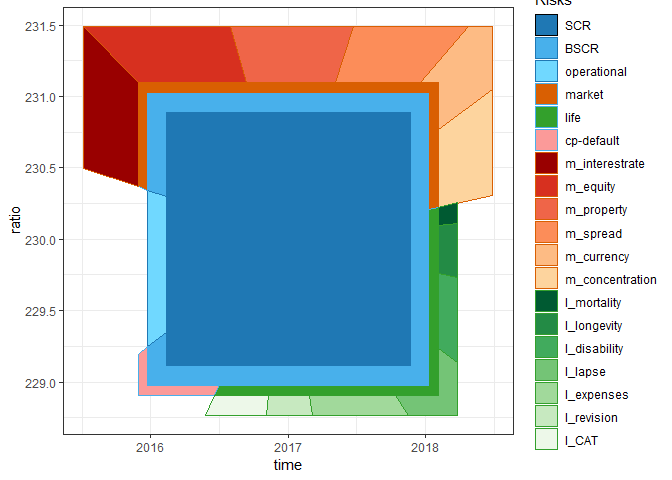# ggsolvencyii 0.1.2## Overview

The goal of ggsolvencyii is to provide ‘ggplot2’-geom’s to show the composition of the Solvency (II) Capital Requirement (SCR) from (diversified) risks and subrisks, whether for standard formula (SF) or (partial) intern models (IM/PIM).

## Installation

You can install ggsolvencyii from Cran (stable release)

``install.packages("ggsolvencyii")``

or (develoment version) from Github:

``````# install.packages("devtools")
devtools::install_github("vanzanden/ggsolvencyii")``````

## short version

This README is a short version of the vignette ‘ggsolvencyii’ at https://cran.r-project.org/web/packages/ggsolvencyii/index.html which shows the same examples with more code. The complete annotated code for the first figure is shown in vignette ‘showcase’. A further elaboration on the inner workings of the package and the use of parameter-values and tables can be found in vignette “coding overview’.

## example

ggsolvencyii builds on ggplot-functionality and provides three geom’s: `geom_sii_risksurface`, `geom_sii_riskoutline` and `geom_sii_riskconnection`.

These three geom’s were used to produce the following showcase plot which might be used for an ORSA (Own Risk and Solvency Assessment) report.

``````#> scaling is based on inputvalue (maxscrvalue) of 25.7433642812936
#> scaling is based on a max (level= 1) value of 25.7433642812936
#> scaling is based on a max (level= 1) value of 25.7433642812936
#> scaling is based on a max (level= 1) value of 25.7433642812936``````The total surface (to the centerpoint of each circle) of the outer segments show the size of undiversified risks. Diversification is made visible by the difference between the risk segment and the next segment nearer to the center of the plot. The red (out)lines are displayed for comparison with the previous SCR buildup.

## Baseplot and options

### base

An life insurer reports its solvency ratio following Standard Formula rules, with English names for all risks. It has a set of risks, diversification effects, and accumulations to the SCR for the current situation (‘id’=1) and two two-year ORSA scenarios. The SCR composition of the current situation (id = 1) is shown below where `sii_z_ex1_data` a tidyverse transformation is of the data.

``````ggplot() +
geom_sii_risksurface(
data = sii_z_ex1_data[sii_z_ex1_data\$id == 1, ],
mapping = aes(x = time, y = ratio, id = id, value = value,
description = description,
fill = description, color = description) ) +
theme_bw() +
scale_fill_manual(name = "Risks",values = sii_x_fillcolors_sf16_eng) +
scale_color_manual(name = "Risks",values = sii_x_edgecolors_sf16_eng)
#> scaling is based on a max (level= 1) value of 23``````### structure

`Geom_sii_risksurface` uses one default table for this plot `sii_structure_sf16_eng`. It defines each risks by indicating from which combined ‘child’-risks and diversification (suffix ‘d’) it is made up. Passing another structure table makes this geom usable for localisation or for internal models.

### levelmax

To prevent cluttering of the legend it is possible to group the smallest items of a level by providing a levelmax-table in such a way that the indicated maximum items in that level is not exceded. the example is on another dataset `sii_z_ex2_data`, with only one SCR result.### Rotation and squared

`rotationdescription` Rotates the plot in such a way that the indicated item (can be on either level, i.e. works also on ‘life’ or ‘operational’) is plotted on just on the righthandside of ‘12 o’clock’. `rotationdegrees’ provides an added rotation. The example rotates ‘m_equity’ to 12 ’o clock, and then 45 degrees counter clockwise.

The option ‘squared’ makes a square plot, with the surface of all segments still in proportion.